﻿ BlackPOM　Spacer(Hexagonal)　/　BS-BE　【Hirosugi-Keiki Co.,Ltd.】

#### POM spacer (Hexagonal black) / BS-BE (Press-fit)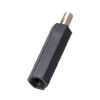【Main body】 Material: Duracon (POM) Color：Black Thermal deformation temperature：1.82Mpa:110℃ UL standard：UL94-HB(Material) 【Male screw】 Material：Free-cut brass(C3604BD)Cadmium content of less than 75 ppm Treatment：Nickel plating(Ni)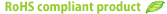■BS-2000BE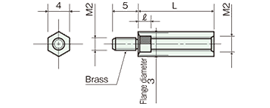Tolerance of L:
±0.1 up to L=15
±0.2 from L=16
ℓ length
3.2 for L=6-7.5
3.7 from L=8
MOQ:50pcs
Screw diameter
(pitch)
L Product code Unit Price(\$)
M2
(0.4)
6 BS-2006BE 0.33
6.5 　BS-2006.5BE 0.33
7 BS-2007BE 0.33
7.5 　BS-2007.5BE 0.33
8 BS-2008BE 0.34
8.5 　BS-2008.5BE 0.34
9 BS-2009BE 0.36
9.5 　BS-2009.5BE 0.36
10 BS-2010BE 0.36
11 BS-2011BE 0.37
12 BS-2012BE 0.37
12.5 　BS-2012.5BE 0.37
13 BS-2013BE 0.37
14 BS-2014BE 0.37
15 BS-2015BE 0.37
16 BS-2016BE 0.38
16.5 　BS-2016.5BE 0.38
17 BS-2017BE 0.38
17.5 　BS-2017.5BE 0.38
18 BS-2018BE 0.38
19 BS-2019BE 0.39
20 BS-2020BE 0.39
21 BS-2021BE 0.41
22 BS-2022BE 0.41
23 BS-2023BE 0.41
24 BS-2024BE 0.42
25 BS-2025BE 0.42
26 BS-2026BE 0.42
27 BS-2027BE 0.44
28 BS-2028BE 0.44
29 BS-2029BE 0.46
30 BS-2030BE 0.46
■BS-2600BE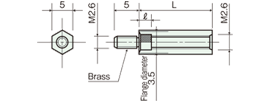Tolerance of L:
±0.1 up to L=15
±0.2 from L=16
ℓ length
3.2 for L=6-7.5
3.7 from L=8
MOQ:50pcs
Screw diameter
(pitch)
L Product code Unit Price(\$)
M2.6
(0.45)
6 BS-2606BE 0.30
6.5 　BS-2606.5BE 0.30
7 BS-2607BE 0.29
7.5 　BS-2607.5BE 0.29
8 BS-2608BE 0.28
.08 　BS-2608.5BE 0.28
9 BS-2609BE 0.28
9.5 　BS-2609.5BE 0.28
10 BS-2610BE 0.28
11 BS-2611BE 0.28
12 BS-2612BE 0.28
12.5 　BS-2612.5BE 0.28
13 BS-2613BE 0.28
14 BS-2614BE 0.30
15 BS-2615BE 0.30
16 BS-2616BE 0.32
16.5 　BS-2616.5BE 0.32
17 BS-2617BE 0.32
17.5 　BS-2617.5BE 0.32
18 BS-2618BE 0.32
19 BS-2619BE 0.33
20 BS-2620BE 0.33
21 BS-2621BE 0.39
22 BS-2622BE 0.39
23 BS-2623BE 0.39
24 BS-2624BE 0.39
25 BS-2625BE 0.39
26 BS-2626BE 0.41
27 BS-2627BE 0.41
28 BS-2628BE 0.41
29 BS-2629BE 0.42
30 BS-2630BE 0.42
■BS-300BE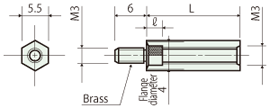Tolerance of L:
±0.1 up to L=15
±0.2 from L=16
ℓ length
3.2 for L=6-7.5
4 from L=8
MOQ:50pcs
Screw diameter
(pitch)
L Product code Unit Price(\$)
M3
(0.5)
6 BS-306BE 0.27
6.5 　BS-306.5BE 0.27
7 BS-307BE 0.27
7.5 　BS-307.5BE 0.27
8 BS-308BE 0.27
8.5 　BS-308.5BE 0.28
9 BS-309BE 0.28
9.5 　BS-309.5BE 0.28
10 BS-310BE 0.28
11 BS-311BE 0.30
12 BS-312BE 0.28
12.5 　BS-312.5BE 0.30
13 BS-313BE 0.28
14 BS-314BE 0.31
15 BS-315BE 0.30
16 BS-316BE 0.31
16.5 　BS-316.5BE 0.31
17 BS-317BE 0.31
17.5 　BS-317.5BE 0.31
18 BS-318BE 0.31
19 BS-319BE 0.32
20 BS-320BE 0.32
21 BS-321BE 0.32
22 BS-322BE 0.32
23 BS-323BE 0.32
24 BS-324BE 0.32
25 BS-325BE 0.34
26 BS-326BE 0.38
27 BS-327BE 0.38
28 BS-328BE 0.38
29 BS-329BE 0.38
30 BS-330BE 0.38
35 BS-335BE 0.39
40 BS-340BE 0.42
45 BS-345BE 0.46
50 BS-350BE 0.48
55 BS-355BE 0.50
60 BS-360BE 0.56
■BS-400BE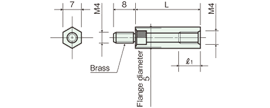Tolerance of L:
±0.1 up to L=15
±0.2 for L=16-100
±0.3 from L=110
MOQ:50pcs
Screw diameter
(pitch)
L Product code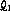Unit Price(\$)
M4
(0.7)
8 BS-408BE 3.8 0.32
9 BS-409BE 4.8 0.32
10 BS-410BE 5.5 0.32
11 BS-411BE 6.5 0.32
12 BS-412BE 7.5 0.32
13 BS-413BE 8.5 0.32
14 BS-414BE 9.5 0.33
15 BS-415BE 10.5 0.33
16 BS-416BE 11.5 0.34
17 BS-417BE 12.5 0.36
18 BS-418BE 13.5 0.36
19 BS-419BE 14.5 0.36
20 BS-420BE 15.5 0.36
21 BS-421BE 16.5 0.38
22 BS-422BE 17.5 0.39
23 BS-423BE 18.5 0.40
24 BS-424BE 19.5 0.40
25 BS-425BE 20.5 0.39
26 BS-426BE 21.5 0.41
27 BS-427BE 22.5 0.42
28 BS-428BE 23.5 0.42
29 BS-429BE 24.5 0.42
30 BS-430BE 25.5 0.41
31 BS-431BE 26.5 0.50
32 BS-432BE 27.5 0.50
33 BS-433BE 28.5 0.50
34 BS-434BE 29.5 0.50
35 BS-435BE 30.5 0.46
36 BS-436BE 31.5 0.52
37 BS-437BE 32.5 0.52
38 BS-438BE 33.5 0.52
39 BS-439BE 34.5 0.52
40 BS-440BE 35.5 0.48
41 BS-441BE 36.5 0.56
42 BS-442BE 37.5 0.56
43 BS-443BE 38.5 0.56
44 BS-444BE 39.5 0.56
45 BS-445BE 40.5 0.53
46 BS-446BE 41.5 0.60
47 BS-447BE 42.5 0.60
48 BS-448BE 43.5 0.60
49 BS-449BE 44.5 0.60
50 BS-450BE 45.5 0.56
51 BS-451BE 46.5 0.63
52 BS-452BE 47.5 0.63
53 BS-453BE 48.5 0.63
54 BS-454BE 49.5 0.63
55 BS-455BE 50.5 0.59
56 BS-456BE 51.5 0.68
57 BS-457BE 52.5 0.68
58 BS-458BE 53.5 0.68
59 BS-459BE 54.5 0.68
60 BS-460BE 55.5 0.59
62 BS-462BE 57.5 0.68
64 BS-464BE 59.5 0.68
65 BS-465BE 60.5 0.70
66 BS-466BE 8 0.74
68 BS-468BE 8 0.74
70 BS-470BE 8 0.76
72 BS-472BE 8 0.79
74 BS-474BE 8 0.79
75 BS-475BE 8 0.78
76 BS-476BE 8 0.88
78 BS-478BE 8 0.88
80 BS-480BE 8 0.87
85 BS-485BE 8 0.90
90 BS-490BE 8 0.93
95 BS-495BE 8 0.98
100 BS-4100BE 8 1.04
110 BS-4110BE 8 1.13
120 BS-4120BE 8 1.20
130 BS-4130BE 8 1.24
140 BS-4140BE 8 1.36
150 BS-4150BE 8 1.47